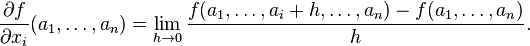# Vector partial derivative

#### cryptoguy

1. The problem statement, all variables and given/known data
A function f(x) : Rn ->R is said to be differentiable at point $$\vec{a}$$ provided that there exists a constant vector $$\vec{c} = (c_1, ... , c_n)$$ such that

$$lim_(\vec{h} -> 0) \frac{f(\vec{a}+\vec{h}) - f(\vec{a}) - \vec{c}*\vec{h}}{||\vec{h}||}$$

Prove that if the multivariable function f(x) (here $$x = x_1, ..., x_n)$$ is differentiable at $$a = (a_1, ..., a_n)$$ then its first order partial derivatives at a exist.
2. Relevant equations
I know that the partial derivative definition is3. The attempt at a solution

I've tried a few things but I've encountered a road block of sorts. I think what I have to do is provide the vector c such that the given equation somehow turns into the definition of the first order partial derivative. That means that instead of having f(a1+h1, a2+h2, ...) I need to make all the h's 0 except one, $$h_i$$ so the term would turn into $$f(a1, a2,..., a_i+h, a_{i+1}...)$$ But I'm not sure how to do that with the vector c... I may be way off base...

Thank you for any hints/advice.

#### Dick

Homework Helper
You want to put $\vec h$ equal to $h \vec {e_i}$. Where e_i is the ith basis vector and take the limit as the real number h approaches 0. Does that help? You can't pick c. That's a given. You can pick a particular form of h.

Last edited:

#### cryptoguy

Thank you for the response. What exactly is an "ith basis vector" though?

#### Dick

Homework Helper
e_i=(0,0,0,...,1,..0,0,0) with the 1 in position i. The same i as in your problem setup.

### The Physics Forums Way

We Value Quality
• Topics based on mainstream science
• Proper English grammar and spelling
We Value Civility
• Positive and compassionate attitudes
• Patience while debating
We Value Productivity
• Disciplined to remain on-topic
• Recognition of own weaknesses
• Solo and co-op problem solving